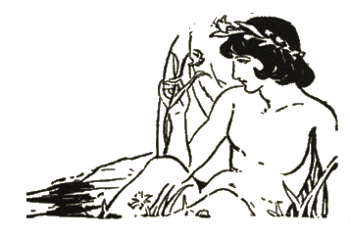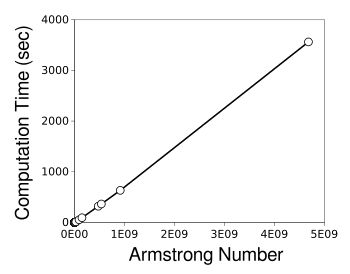### Armstrong Numbers

November 2, 2020

Some believe that vanity is more often found in women than men, but the mythological example of vanity is the male character, Narcissus (Νάρκισσος). Narcissus was a hunter, known for his beauty and vanity, who was punished by the god, Nemesis. Nemesis caused Narcissus to fall in love with his own reflection in a pool of water. Our word, narcissism, comes from this myth.Narcissus, as drawn by Oliver Herford from "An alphabet of celebrities," 1899.

In this drawing, Narcissus is admiring a daffodil, formally known as a Narcissus.

Every time I hear the word, "vanity," I think of the 1972 song, "You're So Vain," by Carly Simon.

(Portion of a Wikimedia Commons image from Project Gutenberg)

Physicists and mathematicians enjoy giving things unusual names. In physics, we have elementary particles with charm, and others that are just strange. In mathematics, there's a number series known as the narcissistic numbers, more formally known as the Armstrong numbers. This sequence is simply defined as m-digit natural numbers equal to sum of the m-th powers of their digits. For example,
153 = 13 + 53 + 33

8208 = 84 + 24 + 04 + 84

4210818 = 47 + 27 + 17 + 07 + 87 + 17 + 87

This is a finite sequence of just 88 elements, the last of which being 115132219018763992565095597973971522401. It could have been called the piano sequence, since a piano has 88 keys, but that might have been confusing, since there's a famous mathematician named Peano. The first few numbers are 1, 2, 3, 4, 5, 6, 7, 8, 9, 153, 370, 371, 407, 1634, 8208, 9474, 54748, 92727, 93084, 548834, 1741725, 4210818, 9800817, 9926315, 24678050, 24678051, and 88593477. Eight of its members end in zero, which ensures that their successors are just one greater.

Although pi can be computed to many digits using a personal computer, the last Armstrong number needs something close to a supercomputer. I modified a code snippet found on the Internet to calculate the Armstrong numbers up to n(32) = 4679307774. You can find my C programming language source code here. As can be seen in the figure, the computation time appears to be linear in n. On my modest dual core 3600 MHz Intel i3-4160 64-bit Linux computer with 8 gigabytes of memory, it took an hour to calculate n(32), so n(88) is clearly impossible on a standard PC. However, harnessing the computing power of one, or more, GPUs might be useful.Computation time for Armstrong numbers on a personal computer having a dual core 3600 MHz Intel i3-4160 64-bit Linux computer with 8 gigabytes of memory.

My Raspberry Pi Model 4B is a factor of 3.6 times slower.

(Graphed using Gnumeric. Click for larger image.)

If we look beyond our ten-finger mindset, we see that Armstrong numbers exist in other number bases, such as base-8 (octal). The number, 432, is 660 in base-8, so we have,
6608 = 63 + 63 + 03
A related sequence, if you're willing to call two numbers a sequence, are the Münchausen numbers, which are the two numbers equal to the sum of their digits raised to the digit's power. These numbers, named after a character in a 1943 film, are 1 and 3435,[4-5]
1 = 11

3435 = 33 + 44 +33 +55 = 27 + 256 + 27 + 3125

If we allow zero to the power of zero to be equal to zero, 00 = 0, as opposed to 00 = 1, we can add 0 and 438579088 to the list.[4-5]

Why are the numbers called Armstrong numbers? They were apparently the result of a homework assignment for a 1966 class in Fortran (at that time, called FORTRAN) at the University of Rochester (Rochester, New York) taught by M.F. Armstrong.An Armstrong of a different kind.